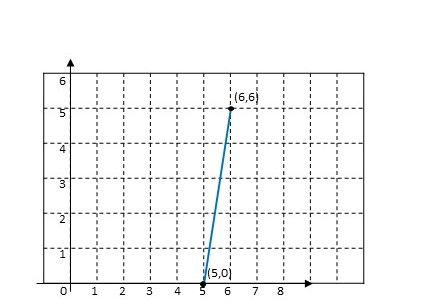# Find the parametric equations that define the curve starting at (5,0) and ending at (6,6), as...

## Question:

Find the parametric equations that define the curve starting at (5,0) and ending at (6,6), as shown. Let parameter t start at 0 and end at 6.

y= _____ (Complete the equation, with the right side in the simplest possible form)

x= _____ (Complete the equation, with the right side in the simplest possible form.## Parametric Curves

A curve in Cartesian coordinates {eq}\displaystyle f(x,y)=0, {/eq} can be written in parametric form by

finding two function of a single parameter that verify the given equation for all values of the parameter, from its domain.

A parametriztion is given as {eq}\displaystyle x=f(t), y=g(t), a\leq t\leq b, {/eq}

If the curve is a line, we will obtain the equation of the line to find a parametrization of the line or line segment.

To find a parametrization for the line segment starting at {eq}\displaystyle (5,0) {/eq} and ending at {eq}\displaystyle (6,6) {/eq}

we will obtain the equation of the line passing through the two points,

{eq}\displaystyle y=6(x-5). {/eq}

To start the parametrization at {eq}\displaystyle t=0, {/eq} we will use {eq}\displaystyle y=t \text{ and } x=\frac{y+30}{6}=5+\frac{t}{6}. {/eq}

So, a parametrization of the line segment is given as {eq}\displaystyle \boxed{x=5+\frac{t}{6}, y=t, 0\leq t\leq 6}. {/eq}